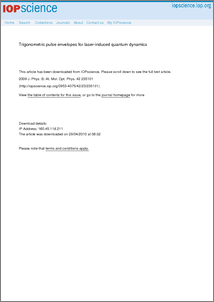Repository: Freie Universität Berlin, Math Department

# Trigonometric pulse envelopes for laser-induced quantum dynamics

Barth, I. and Lasser, C. (2009) Trigonometric pulse envelopes for laser-induced quantum dynamics. J. Phys. B: At. Mol. Opt. Phys., 42 (23). p. 235101.Preview

347kB

Official URL: http://dx.doi.org/10.1088/0953-4075/42/23/235101

## Abstract

We relate powers of trigonometric functions to Gaussians by proving that properly truncated cosn functions converge to a Gaussian as n tends to infinity. For an application, we analyse the laser-induced population transfer |X1Σ+ → |A1Πx in a two-level model system of aluminium monochloride (AlCl) with fixed nuclei. We apply linearly x-polarized ultraviolet laser pulses with a trigonometric envelope function, whose square has full width at half-maximum of 2.5 fs and 5.0 fs. Studying population dynamics and optimized laser parameters, we find that the optimal field amplitude for trigonometric pulses with n = 20 and n = 1000 has a relative difference of 1%, which is below experimental resolution.

Item Type: Article Physical Sciences > Physics > Mathematical & Theoretical Physics > Quantum Mechanics Department of Mathematics and Computer Science > Institute of Mathematics 899 Burkhard Schmidt 29 Apr 2010 07:36 03 Mar 2017 14:40

Repository Staff Only: item control page